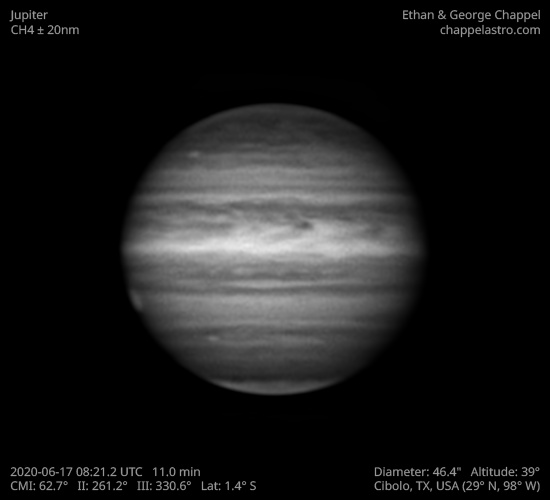# Jupiter 2020-06-17 08:21 UTC

CM1: 62.70°

CM2: 261.20°

CM3: 330.60°

CLat: -1.40°

Description

A second morning of fairly good seeing. It turns out the root of my motor focus problems was the serial cable connecting it to the controller. A new one was supposed to arrive a couple days ago, but the envelope was empty because it was improperly sealed!

Notes:

• The NEB is thinner on this hemisphere.
• The Great Red Spot is rising.

Equipment

ZWO ASI290MM

Celestron EdgeHD 14

Logs
```FireCapture v2.6  Settings
------------------------------------
Observer=Ethan Chappel
Location=Cibolo
Scope=Celestron C14 EdgeHD
Camera=ZWO ASI290MM
Filter=CH4
Profile=Jupiter
Diameter=46.29"
Magnitude=-2.66
CMI=64.3° CMII=262.8° CMIII=332.3°  (during mid of capture)
FocalLength=3450mm (F/9)
Resolution=0.17"
Filename=2020-06-17-0824_2-EC-CH4-Jup.ser
Date=2020_06_17
Start=08_21_46.111
Mid=08_24_16.140
End=08_26_46.170
Start(UT)=08_21_46.111
Mid(UT)=08_24_16.140
End(UT)=08_26_46.170
Duration=300.059s
Date_format=yyyy_MM_dd
Time_format=HH_mm_ss
LT=UT -6h
Frames captured=5001
File type=SER
Binning=no
ROI=404x354
ROI(Offset)=0x0
FPS (avg.)=16
Shutter=60.00ms
Gain=470 (78%)
SoftwareGain=10 (off)
AutoHisto=75 (off)
Brightness=1 (off)
Gamma=50 (off)
USBTraffic=50 (off)
AutoGain=off
FPS=100 (off)
AutoExposure=off
HighSpeed=on
Histogramm(min)=0
Histogramm(max)=178
Histogramm=69%
Noise(avg.deviation)=4.68
Limit=300 Seconds
Sensor temperature=28.6°C
FireCapture v2.6  Settings
------------------------------------
Observer=Ethan Chappel
Location=Cibolo
Scope=Celestron C14 EdgeHD
Camera=ZWO ASI290MM
Filter=CH4
Profile=Jupiter
Diameter=46.29"
Magnitude=-2.66
CMI=60.6° CMII=259.2° CMIII=328.6°  (during mid of capture)
FocalLength=3450mm (F/9)
Resolution=0.17"
Filename=2020-06-17-0818_2-EC-CH4-Jup.ser
Date=2020_06_17
Start=08_15_42.284
Mid=08_18_12.287
End=08_20_42.291
Start(UT)=08_15_42.284
Mid(UT)=08_18_12.287
End(UT)=08_20_42.291
Duration=300.007s
Date_format=yyyy_MM_dd
Time_format=HH_mm_ss
LT=UT -6h
Frames captured=5000
File type=SER
Binning=no
ROI=404x354
ROI(Offset)=0x0
FPS (avg.)=16
Shutter=60.00ms
Gain=470 (78%)
SoftwareGain=10 (off)
AutoHisto=75 (off)
Brightness=1 (off)
Gamma=50 (off)
USBTraffic=50 (off)
AutoGain=off
FPS=100 (off)
AutoExposure=off
HighSpeed=on
Histogramm(min)=0
Histogramm(max)=174
Histogramm=68%
Noise(avg.deviation)=4.71
Limit=300 Seconds
Sensor temperature=29.0°C
```